This activity is related to a Teachable Moment from Oct. 31, 2016. See "When Computers Were Human."

› Explore more on the Teachable Moments Blog

### Overview

This activity is designed for students familiar with advanced algebra concepts. In this lesson, students will:

• Use algebraic computations to determine the relative positions of Earth and Mars during which an optimal (low-energy) transfer of a spacecraft can occur.
• Combine this information with planetary-position data to determine the next launch opportunity to Mars.

### Management

Consider having students sit on a carpeted floor when using the pushpins and string to make an ellipse. The carpeted floor will absorb the tips of the pushpins that may extend past the thickness of the cardboard. Alternately, have students use more than one piece of cardboard to cushion against protruding pushpin tips.

### Background

When a spacecraft is launched from Earth, its forward velocity combined with the gravitational pull of Earth cause it to travel in a curved path. As the spacecraft heads toward another planet, the gravitational pull of that planet factors in to the path the spacecraft takes. The more a spacecraft can “coast” with engines off, the lower the cost of the mission (rocket fuel is not cheap!).

Think of a quarterback throwing a football to a receiver. The initial impulse (throw) is all the football gets as far as power is concerned. The football follows a curved path into the hands of the receiver. Likewise, the quarterback throws the football to where the receiver is going to be, not necessarily to where the receiver is currently. So, the quarterback throws the football downfield as the receiver is running in that direction. In a perfectly thrown pass, the receiver’s running speed will bring him or her to the exact spot where the football arrives at hand-level.

Launching to Mars is similar to this. A spacecraft is given an initial impulse (launch) toward Mars and then shuts off its engines and coasts (obeying Newton’s First Law) until it gets close to its target. Depending on the mission, the spacecraft may slow down – to get into orbit or land – by using the Martian atmosphere or retro-rockets that fire opposite to the direction of travel (obeying Newton’s Third Law).

Though a spacecraft could follow a variety of curved paths from Earth to Mars, one path called the Hohmann transfer orbit uses the least energy and is thereby considered to be the most efficient.

The Hohmann transfer is an elliptical orbit with the sun at one focus of the ellipse that intersects the orbit of the target planet. Launch occurs when Earth is at Hohmann perihelion (the point of the Hohmann orbit that is closest to the sun). Arrival occurs when Mars is at Hohmann aphelion (the point of the Hohmann orbit that is farthest from the sun).

Depending on mission objectives and spacecraft characteristics, engineers will use variations on the Hohmann transfer orbit to get spacecraft to Mars. These variations can make travel time more or less lengthy than a standard Hohmann transfer.

To make sure the spacecraft and Mars arrive at the same place at the same time, the spacecraft must launch within a particular window of time. This window is called the “launch window” and, depending on the target, can be a few minutes or as much as a few weeks in length.

If a spacecraft is launched too early or too late, it will arrive in the planet’s orbit when the planet is not there.When launched within the proper launch window, the spacecraft will arrive in the planet’s orbit just as the planet arrives at that same place. At this point, the spacecraft is positioned for either going into orbit about the planet or landing on the planet.Calculating orbit trajectories and launch windows is a complex task involving a variety of parameters that may or may not be constantly changing. In order to make this task accessible to high-school students, some variable parameters have been stabilized and some assumptions have been made. This problem, with these simplifications, allows students to generate an approximation of the launch window to Mars.

### Procedures

1. Explain to students that launching to Mars requires a spacecraft to travel in an elliptical orbit about the sun such that the spacecraft and Mars will arrive in the same place at the same time. Their task in this exercise is to determine when we should next launch to Mars.

2. Explain that the most energy efficient orbit of this type is called the Hohmann transfer, in which the spacecraft will travel half of one orbit about the sun, leaving Earth at the orbit’s perihelion and arriving at Mars (or any outer planet) at the orbit's aphelion.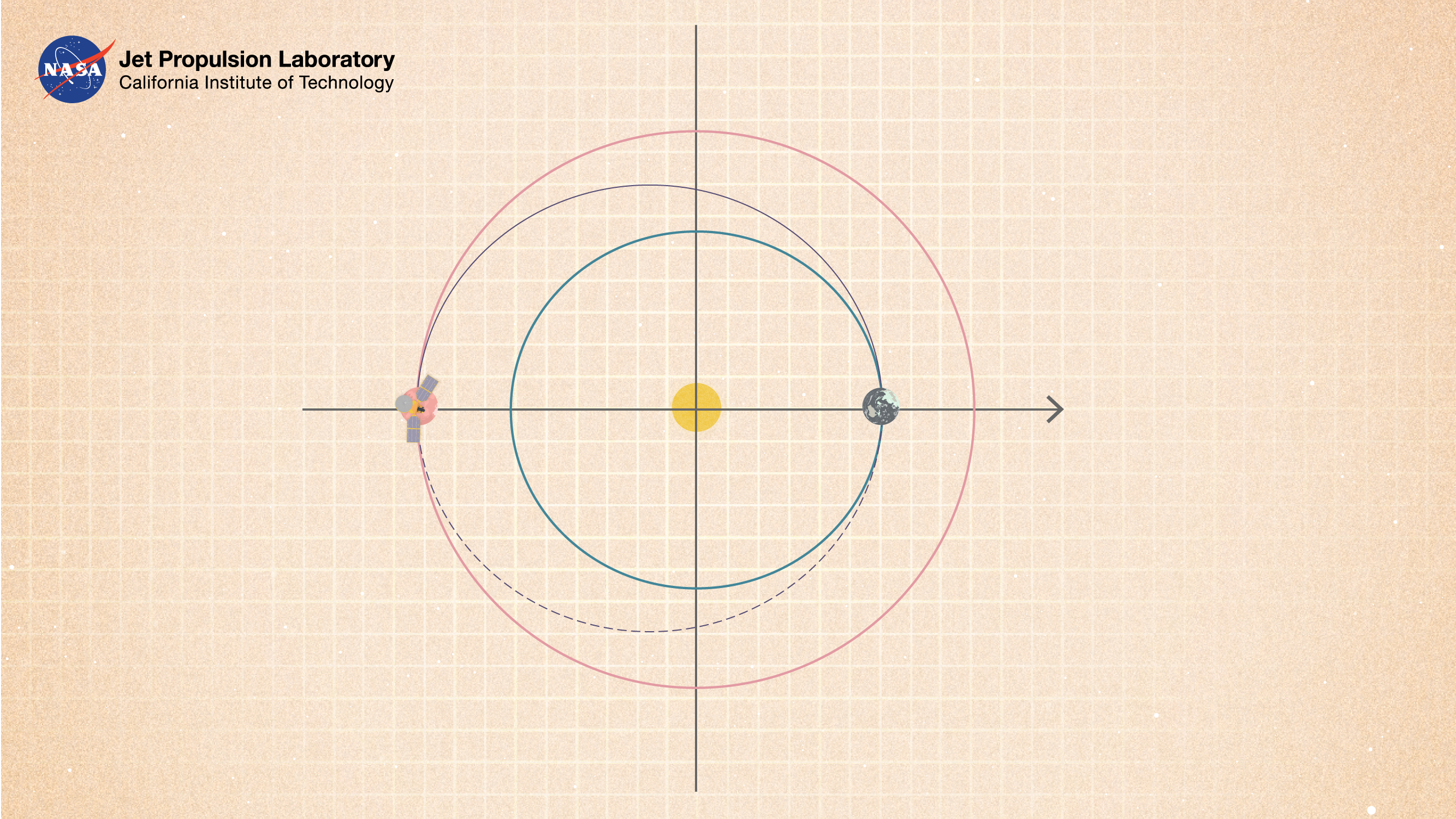The red line indicates the orbit of Mars, the blue line indicates the orbit of Earth, and the grey line indicates the path a spacecraft takes from Earth to Mars when launched on a Hohmann transfer path.

3. Remind students of Kepler’s Second Law, the Law of Equal Areas: A line drawn from a planet to the sun sweeps out equal areas in equal amounts of time.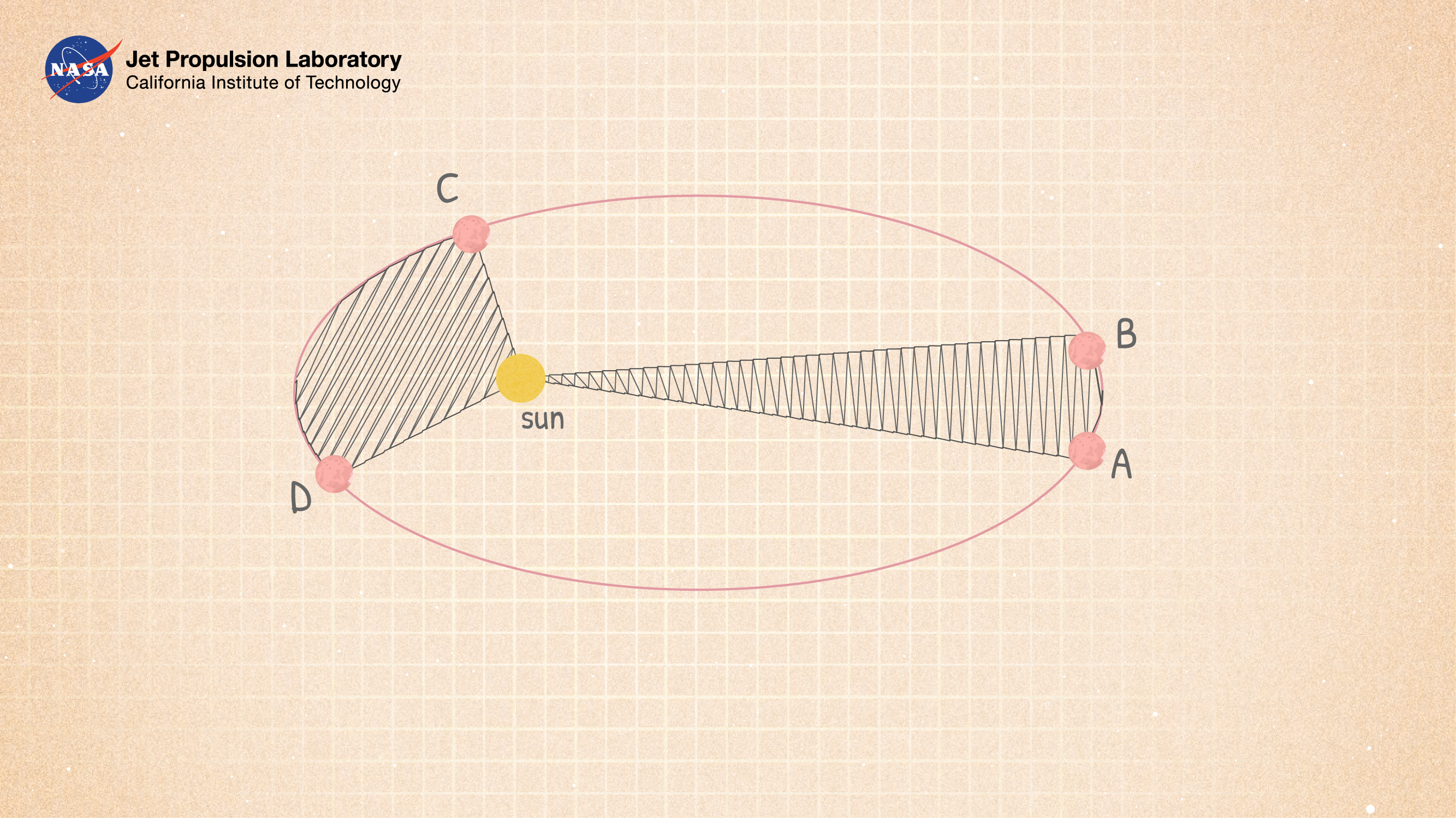Kepler's Second Law also tells us that planets travel at different rates of speed in their elliptical orbits, moving faster when they are closer to the sun and slower when they are farther from the sun.

4. Explain to students that launching a spacecraft while considering the orbital dynamics of the planets is a highly complex mathematical task. In order to simplify the task, we will make three assumptions (Note: none of these assumptions are true, but using these simplifications will still allow a fairly accurate computation of the launch window.):
• The orbits of Earth and Mars are circular and centered on the sun. (Earth’s orbit is more circular than Mars’ orbit, but they are both slightly elliptical.)
• Earth and Mars travel at constant speeds. (They do not. See Kepler’s Second Law).
• The orbits of Earth and Mars are in the same plane. (They are close but slightly out of plane with one another).

5. Explain to students the concept of heliocentric longitude. This is the position of an object with respect to the sun, measured eastward along the ecliptic (path of Earth around the sun) from the vernal equinox (position in space where the ecliptic crosses the celestial equator). Just as longitudes on Earth measure position with respect to a fixed point (the prime meridian), heliocentric longitudes measure position in space along the ecliptic with respect to the vernal equinox.For consistency, we measure heliocentric longitudes counterclockwise (as viewed from above) from the vernal equinox.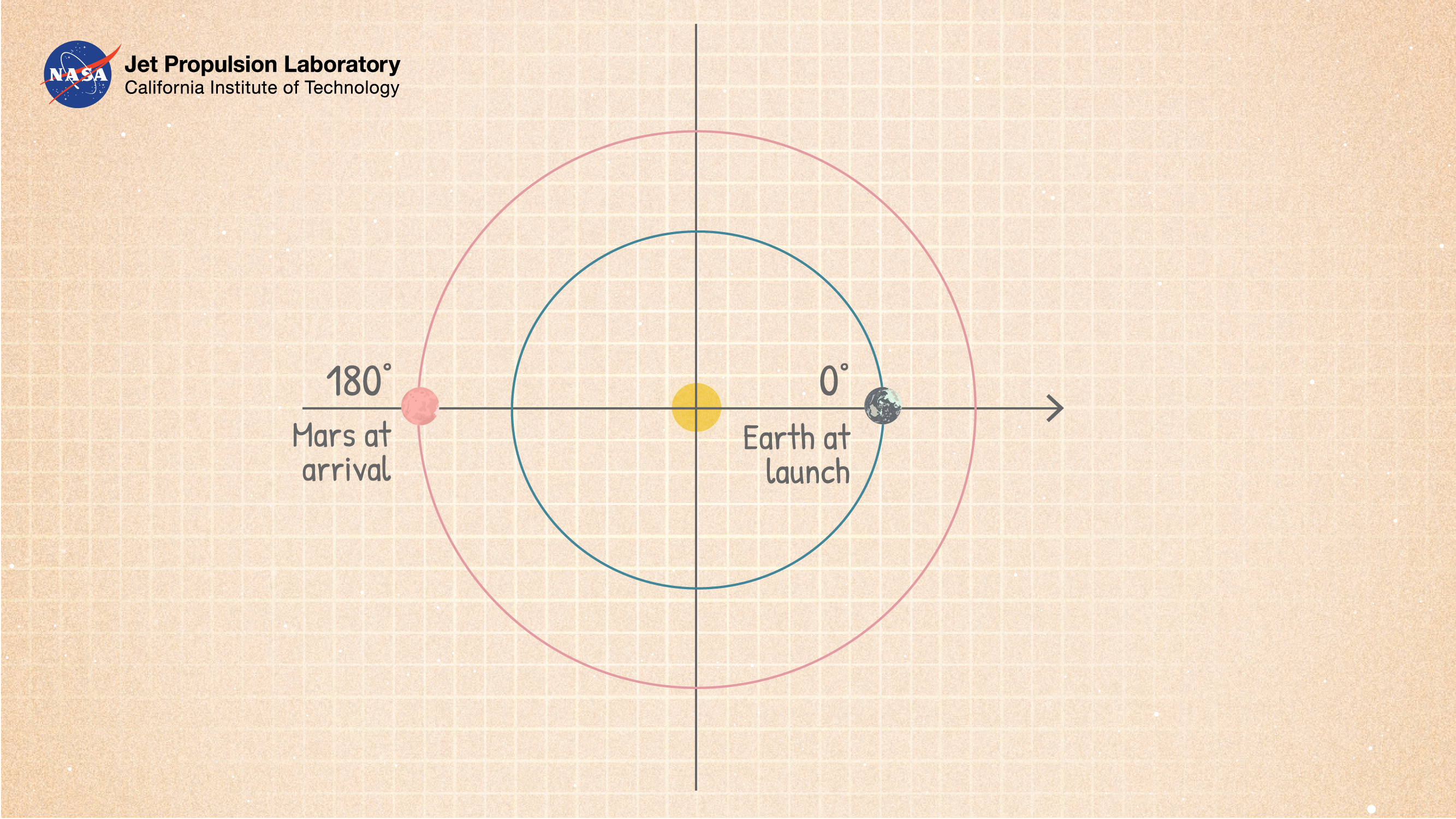To establish a frame of reference for this problem, we place Earth at launch at the vernal equinox (0 degrees) and Mars at 180 degrees at arrival.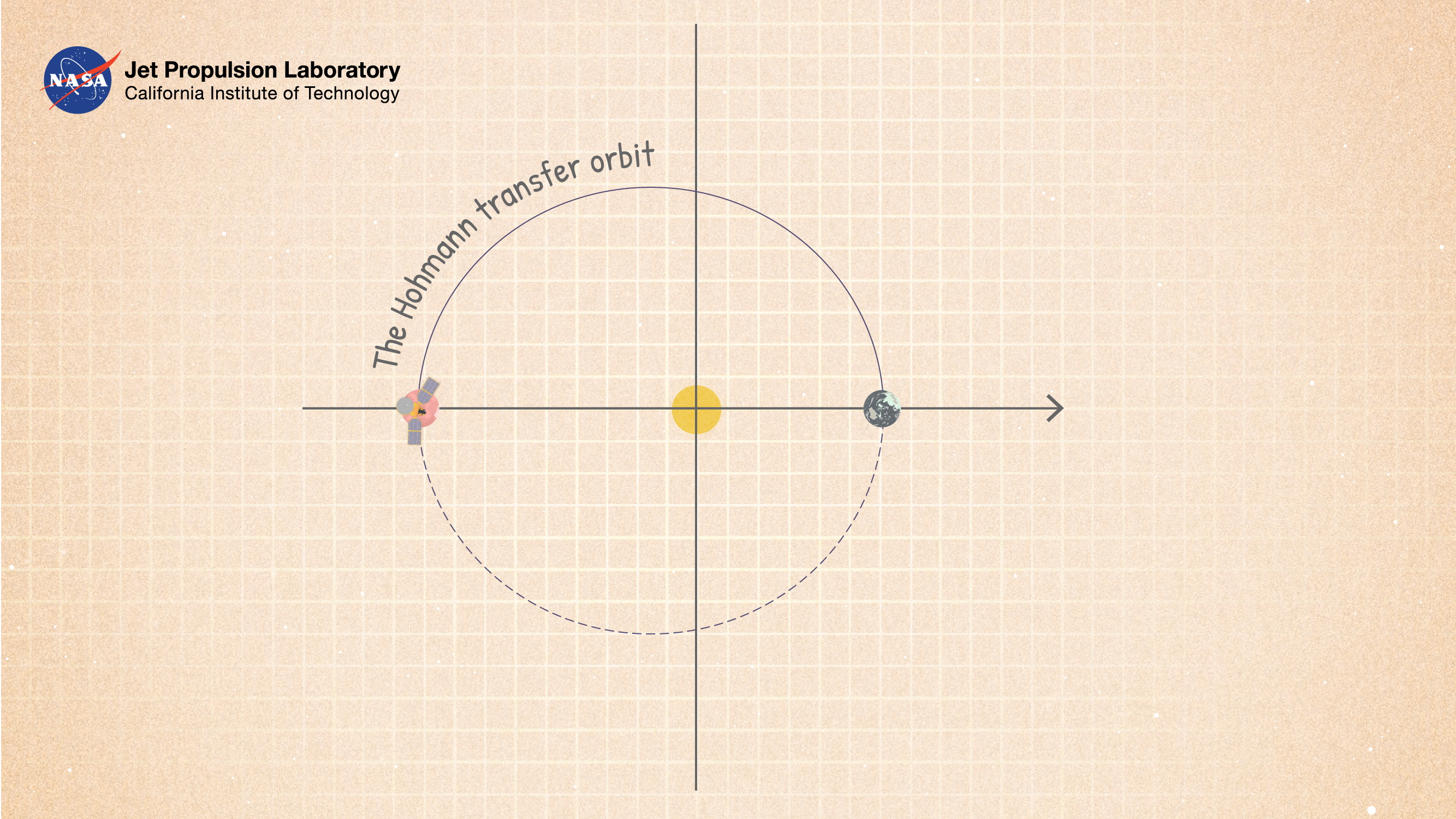The Hohmann transfer orbit is the ellipse that connects the points in space, Earth at 0 degrees and Mars at 180 degrees, about the ellipse that has the sun at one focus.

6. Have students find the length of the semi-major axis of the transfer orbit in astronomical units (AU), given that the average distance from Mars to the sun is 1.52 AU.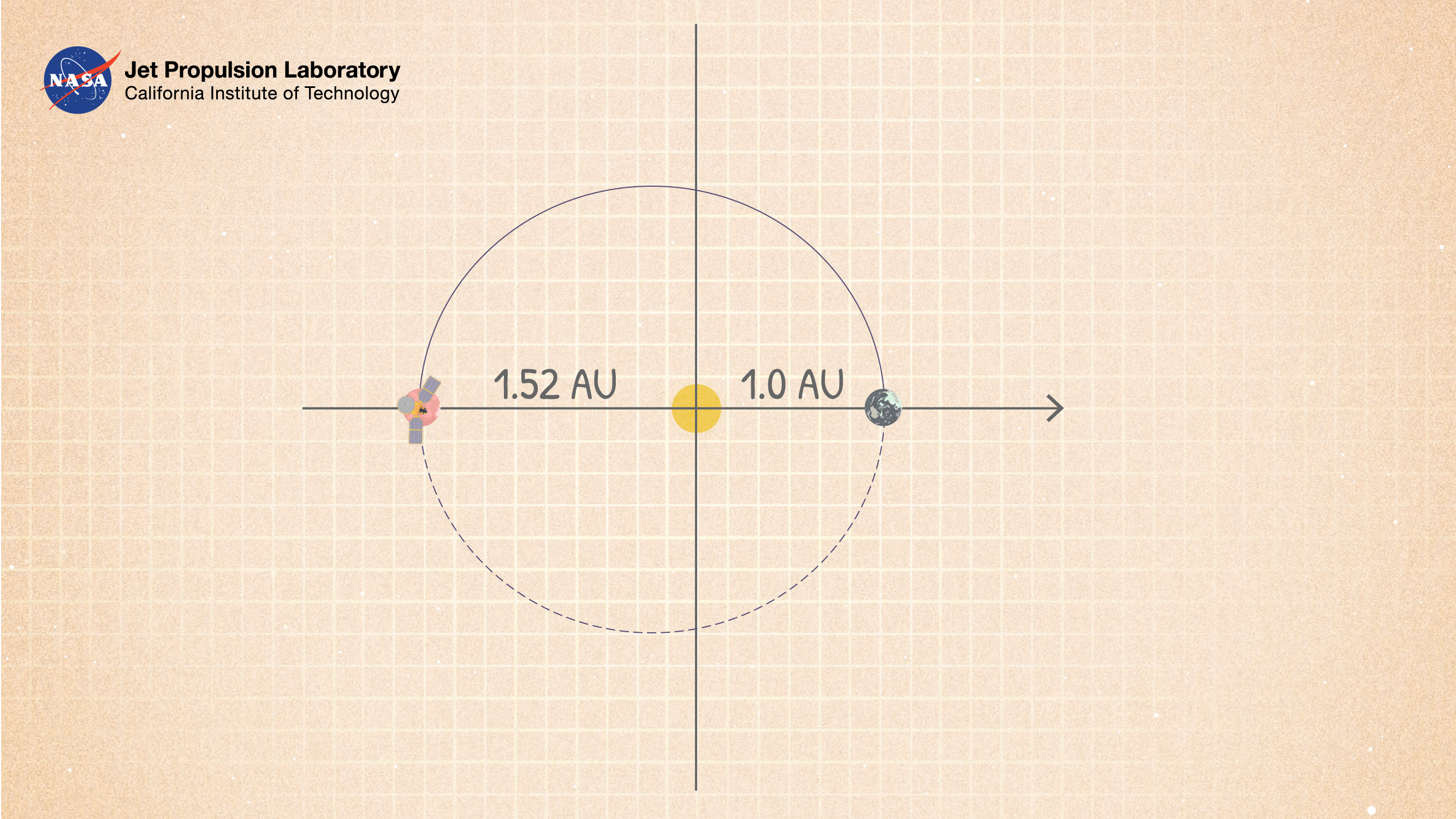Earth is, on average, 1 astronomical unit (AU) from the sun. Mars is, on average, 1.52 AUs from the sun.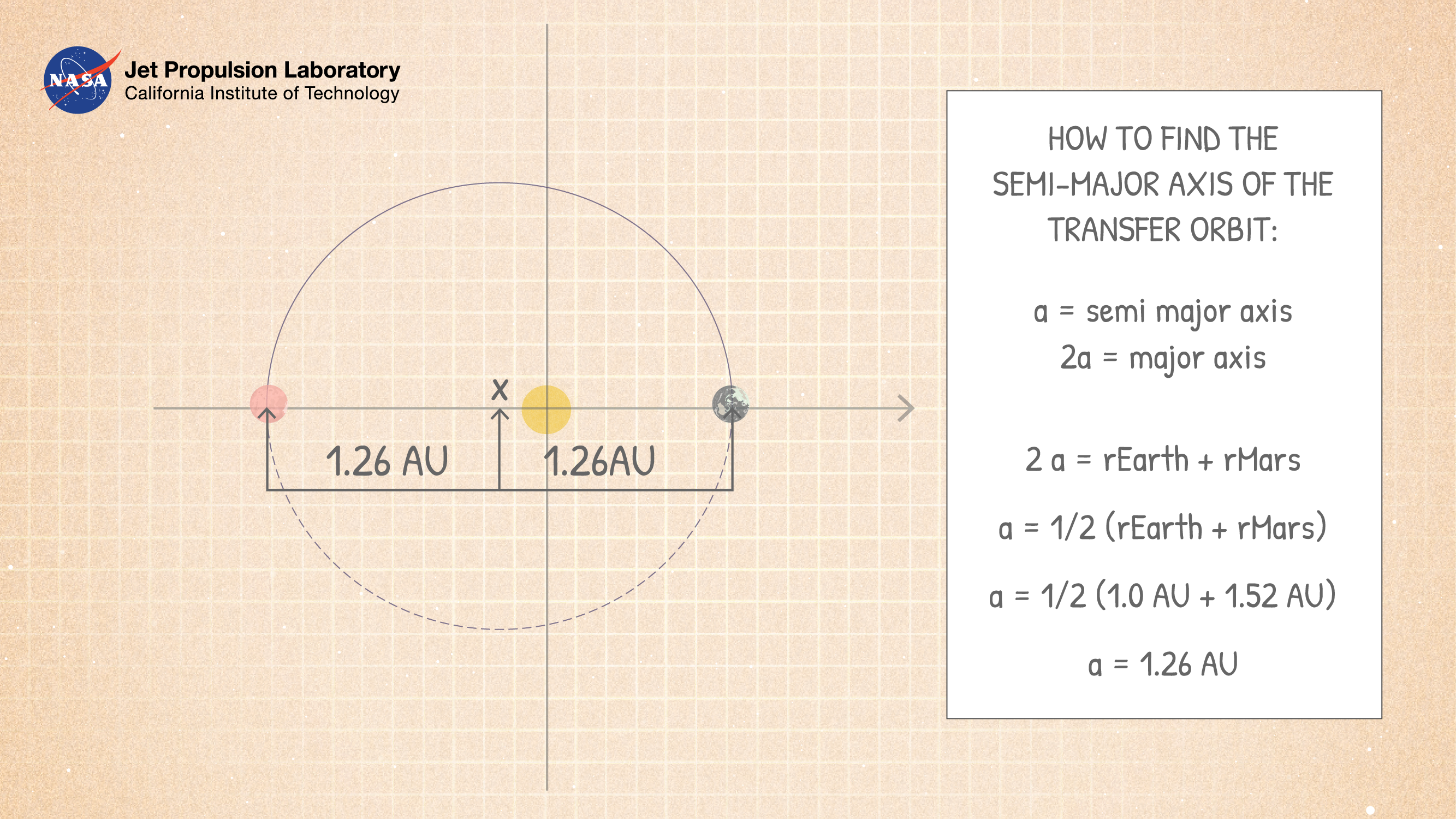The major axis of the Hohmann transfer orbit is represented by 2a. Some simple arithmetic will allow us to compute the length, a, of the semi-major axis.

7. Have students use string and pushpins to draw the assumed-circular orbits of Earth and Mars about the sun, and the approximation of the Hohmann transfer orbit on graph paper as shown at right:

Students will need to compute the location of the second focus (one focus is at the sun) for the Hohmann transfer orbit. The focal distance is 0.26 AU, so if the sun is at (0,0), the other focus will be at (-0.52, 0).Use string and two pushpins to draw the elliptical Hohmann transfer orbit.

To draw the Hohmann transfer orbit, place a pushpin at each focus of the ellipse and use a loop of string equal in length to twice the sum of the length of the semi-major axis of the ellipse and the focal length (students may derive this using the formula for an ellipse).

8. Have students use Kepler’s Third Law, the Law of Harmony, to determine the period of the Hohmann transfer orbit and then the travel time to Mars along this orbit.

Kepler’s Third Law states that the square of the period of any planet is proportional to the cube of the semi-major axis of its orbit. An equation can represent this relationship:

P2=ka3 with k being the constant of proportionality

Using Earth as an example, we can measure P in years and a in astronomical units so P = 1 year and a = 1 AU. Thus, P2=ka3→k=1 => P2=a3

P2= (1.26 AU)3 => P ~ 1.41 years ~ 517 days

The full period of this Hohmann transfer orbit is 517 days. Travel to Mars encompasses half of one orbit, so approximately 259 days.

9. Using the daily motions of Earth and Mars, compute the ideal relative position of Earth and Mars during launch.

Mars completes one revolution around the sun (360 degrees) in 687 days, so that means it moves 0.524 degrees per day (360 degrees/687 days). In 259 days (the travel time from Earth to Mars along the Hohmann transfer path), Mars will have moved 136 degrees (0.524 degrees per day * 259 days).

To calculate the position of Mars at the time of launch, subtract the amount of its motion during the spacecraft’s travel time (136 degrees) from its point of arrival (180 degrees). 180 degrees – 136 degrees = 44 degrees.

Considering that launch from Earth was at the Hohmann orbit perihelion (point closest to the sun) and arrival is at the Hohmann orbit aphelion (point farthest from the sun), we can conclude that a launch opportunity occurs when Mars is 44 degrees ahead of Earth in its orbit.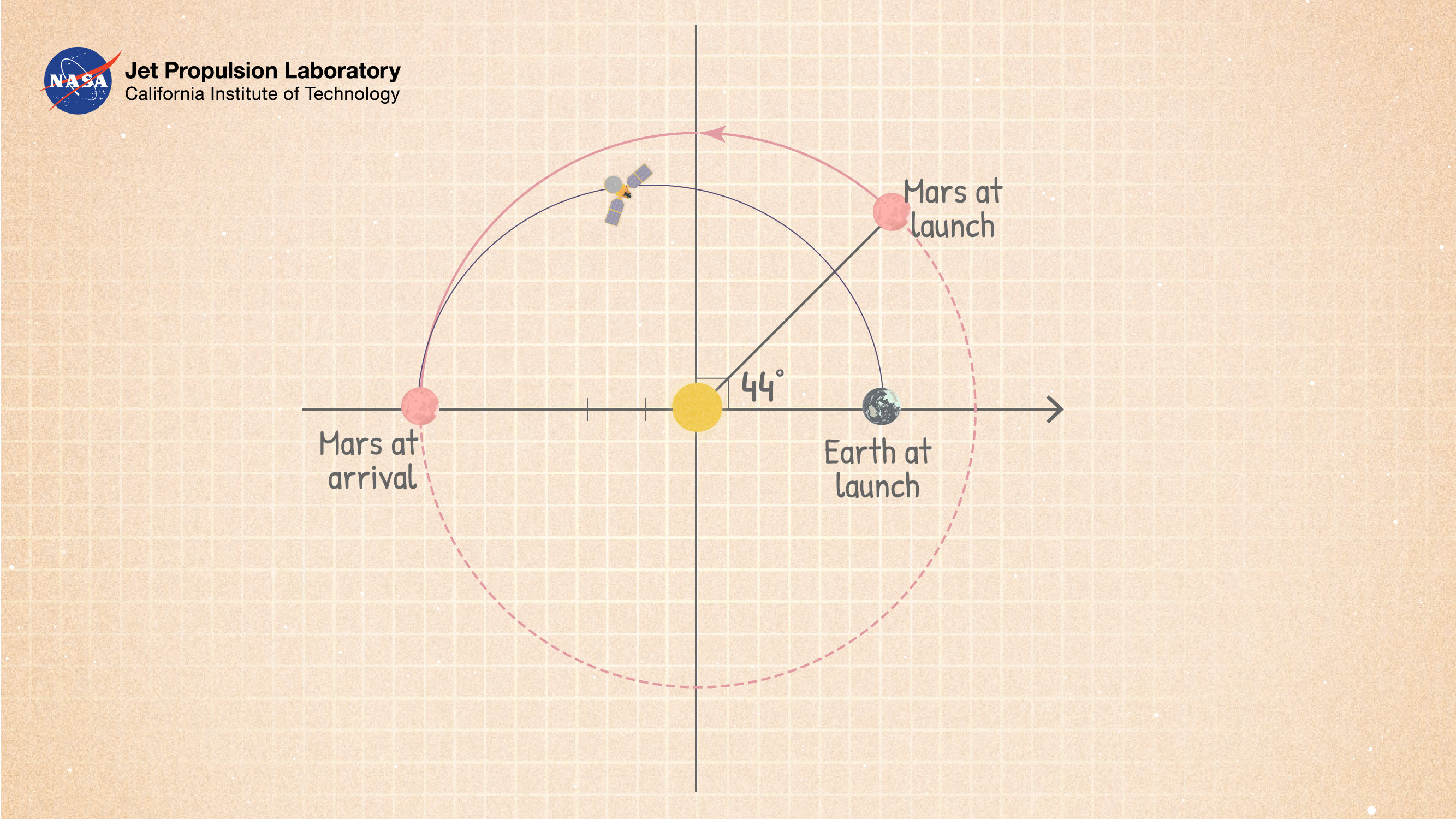In our established frame of reference, Mars must be at 44 degrees relative to Earth at 0 degrees at launch. For any frame of reference, Mars must be 44 degrees ahead of Earth in its orbit at launch.

10. Using the planetary heliocentric longitudes, approximately when is the next opportunity for a launch to Mars?

### Discussion

• Must a spacecraft be launched at an exact moment in the launch window? What happens if it is launched early or late?
• Research: What is the average length of a launch window to Mars?

### Extensions

• Approximately when was the most recent opportunity for a launch to Mars? What countries took advantage of that opportunity and launched to Mars at that time? What is the current status of those missions? Were they successful?
• Have students create a spreadsheet that will subtract heliocentric longitudes for Earth and Mars to simplify launch window calculations.
• Relative to Mars, where is Earth in its orbit when the spacecraft arrives?